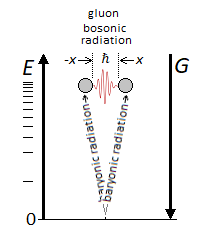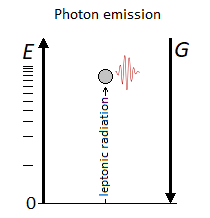# Vertical Oscillation of the Particle following the Gravitational Force Vector

## Recommended Posts

I made a naming mistake by using the word leptonic instead of baryonic for the previous diagram. Nothing contemptuous. If not that it should be emphasized. I therefore reiterate the message with the correct diagram:

If the leptonic/baryonic matter is indeed radiative and vertical to gravity, then the bosons are only the difference in horizontal potential between two oscillations of the particle. It reminds me of the photon emitted by the oscillation of the electron.We understand that the particle collapses at any time thanks to gravity, and reappears thanks to energy.

I take this opportunity to publish the leptonic oscillation with the following diagram:Vertical radiation = Fermionic matter = particle
Horizontal radiation = Bosonic matter = wave

I wish to continue with the Heisenberg's uncertainty principle for macroscopic objects of the order of millimeter. I recall the initial conditions that I wish:

∆x corresponds to the width of the particle at rest at h, that is E=0. For the moment we only have one exploitable interval height which is (H-h), H being the maximum height that the particle can reach. The particle therefore oscillates vertically from h to H and from H to h at a constant* width of ∆x. Also the impulse is constant and corresponds to the force of energy necessary to equal in terms of compensation the inverse speed of the classical gravitational acceleration (1 g = 9.806 65 m s − ²) ; Or quantum? Namely the gravitational wave at the speed of light. Everything is experienced in a vacuum.

The units are meter, second, and kilogram.

$${\displaystyle \\ mv\ =\ \sqrt{2m^2g(H-h)}\ \\ v\ =\ \sqrt{2g(H-h)}\ \\ \\}$$

$$\\ \Delta v\ =\ \sqrt{2g(H-h2)}\ -\ \sqrt{2g(H-h1)} \\ \\ \Delta x\ =\ (x2-x1)\ = 10 ^- 3 \\ \\$$

$$\\ \Delta x\Delta v\ \ {\ge } \ {\frac{\hbar}{2m}}$$

$$and:$$
$$\\ \Delta p\ =\ \sqrt{2m^2g(H-h2)}\ -\ \sqrt{2m^2g(H-h1)} \\ \\ \Delta x\Delta p\ \ {\ge } \ {\frac{\hbar}{2}}$$

* The question is and with the principle of uncertainty applied: will the particle oscillate transversely along x to give a value greater than ∆x?

Edited by Kartazion
##### Share on other sites

On 7/9/2021 at 6:47 PM, Kartazion said:

I only said the unit was meter.

You said x=1 and the unit was a meter

On 7/9/2021 at 6:47 PM, Kartazion said:

After I specified 1 mm or 1 nm at your choices for the particle or quanta. We can also talk about 1cm. Indeed the interval Delta is x2=0,5 and x1=-0,5. But what are the different situations? You mean the principle of uncertainty which applies more or less depending on the size of the mass? This is true, because for macroscopic objects the principle of uncertainty is negligible.

The uncertainty principle is not relevant to the question of the OP

On 7/9/2021 at 6:47 PM, Kartazion said:

You have a width of 1 for x and y which oscillates more than 1 given the height. Which makes two dimensions with a surface x*y.

You said it again. Width of 1

On 7/9/2021 at 6:47 PM, Kartazion said:

However, it is simple if the observer is at the top at H there will be an oscillation of the particle in the form of a magnitude. As simple as that.

“an oscillation of the particle in the form of a magnitude” is a meaningless phrase, and has no relevance to the OP

On 7/9/2021 at 6:47 PM, Kartazion said:

But then what is the unit of x here in Heisenberg's formula?

ΔxΔv   2m

The HUP is a separate topic. Open a new thread. This was started as a discussion of a classical physics problem of a particle falling under gravity

1 hour ago, Kartazion said:

Nobody?

You’re spouting nonsense, so what do you expect?

25 minutes ago, Kartazion said:

I wish to continue with the Heisenberg's uncertainty principle for macroscopic objects of the order of millimeter. I

!

Moderator Note

##### Share on other sitesThis topic is now closed to further replies.
×